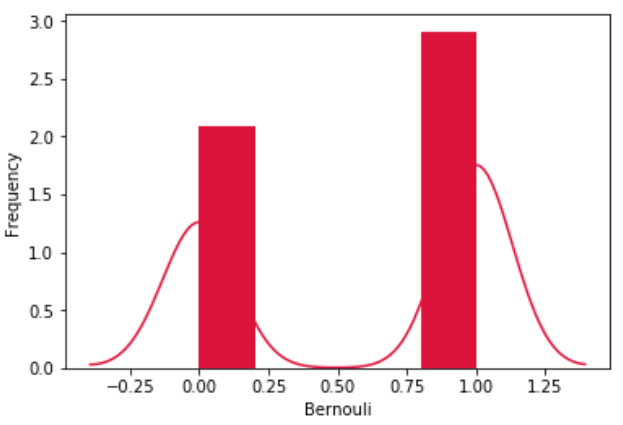# Python – Bernoulli Distribution

The Bernoulli distribution is a special case of the Binomial distribution where a single experiment is conducted so that the number of observation is 1. So, the Bernoulli distribution therefore describes events having exactly two outcomes.

We use various functions in numpy library to mathematically calculate the values for a bernoulli distribution. Histograms are created over which we plot the probability distribution curve.

```from scipy.stats import bernoulli
import seaborn as sb

data_bern = bernoulli.rvs(size=1000,p=0.6)
ax = sb.distplot(data_bern,
kde=True,
color='crimson',
hist_kws={"linewidth": 25,'alpha':1})
ax.set(xlabel='Bernouli', ylabel='Frequency')```

Its output is as follows −